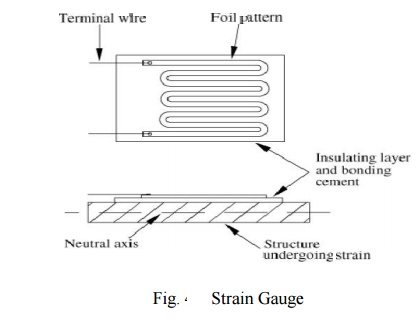# Strain gauge: Principle of Working, Materials Used, ApplicationsStrain gauges are devices whose resistance changes under the application of force or strain. They can be used for measurement of force, strain, stress, pressure, displacement, acceleration etc.

Strain gauge

Strain gauges are devices whose resistance changes under the application of force or strain. They can be used for measurement of force, strain, stress, pressure, displacement, acceleration etc.

## What are Strain Gauges?

It is often easy to measure the parameters like length, displacement, weight etc that can be felt easily by some senses. However, it is very difficult to measure the dimensions like force, stress and strain that cannot be really sensed directly by any instrument. For such cases special devices called strain gauges are very useful.

There are some materials whose resistance changes when strain is applied to them or when they are stretched and this change in resistance can be measured easily. For applying the strain you need force, thus the change in resistance of the material can be calibrated to measure the applied force. Thus the devices whose resistance changes due to applied strain or applied force are called as the strain gauges.

## Principle of Working of Strain Gauges

When force is applied to any metallic wire its length increases due to the strain. The more is the applied force, more is the strain and more is the increase in length of the wire. If L 1 is the initial length of the wire and L2 is the final length after application of the force, the strain is given as: ε = (L2-L1)/L1 Further, as the length of the stretched wire increases, its diameter decreasesNow, we know that resistance of the conductor is the inverse function of the length. As the length of the conductor increases its resistance decreases. This change in resistance of the conductor can be measured easily and calibrated against the applied force. Thus strain gauges can be used to measure force and related parameters like displacement and stress. The input and output relationship of the strain gauges can be expressed by the term gauge factor or gauge gradient, which is defined as the change in resistance R for the given value of applied strain ε.

## Materials Used for the Strain Gauges

Earlier wire types of strain gauges were used commonly, which are now being replaced by the metal foil types of gauges as shown in the figure below. The metals can be easily cut into the zigzag foils for the formation of the strain gauges. One of the most popular materials used for the strain gauges is the copper-nickel-manganese alloy, which is known by the trade name ‘Advance.’ Some semiconductor materials can also be used for making the strain gauges.

## Applications of the Strain Gauges

The strain gauges are used for two main purposes:

1) Measurement of strain: Whenever any material is subjected to high loads, they come under strain, which can be measured easily with the strain gauges. The strain can also be used to carry out stress analysis of the member.

2) Measurement of other quantities: The principle of change in resistance due to applied force can also be calibrated to measure a number of other quantities like force, pressure, displacement, acceleration etc since all these parameters are related to each other. The strain gauges can sense the displacements as small as 5 µm. They are usually connected to the mechanical transducers like bellows for measuring pressure and displacement and other quantities.

Study Material, Lecturing Notes, Assignment, Reference, Wiki description explanation, brief detail

Related Topics